Equivalent Fractions Worksheet

Equivalent Fractions Worksheets

Fraction Circles

Fraction Worksheets

Equivalent Fractions – Equivalent Fractions Example – Equivalent Fractions Meaning

Equivalent Fractions -Equivalent fractions are equal to each other. Two fractions are equal if they represent the same portion of a whole.

For example,

1/2=2/4=4/8

and

1/3=2/6=4/12

Equivalent Fractions With Pictures

Find the equivalent fractions.

### New Worksheets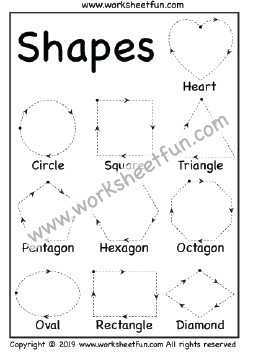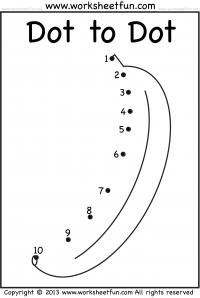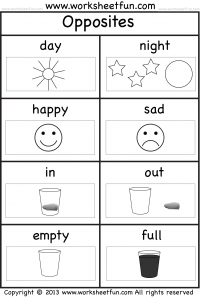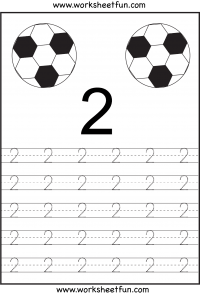### Most Popular Preschool and Kindergarten Worksheets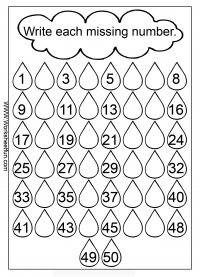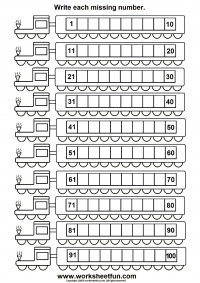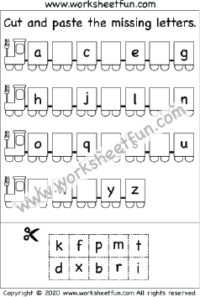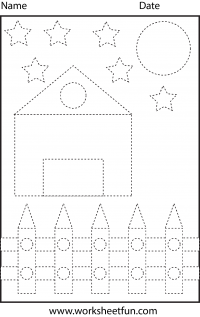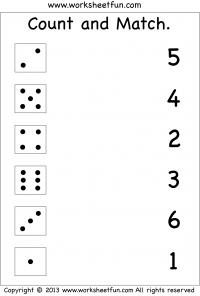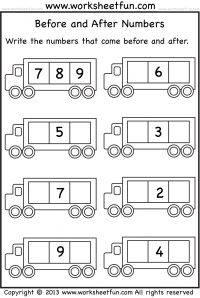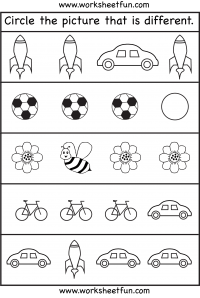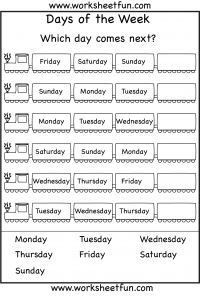#### Kindergarten Worksheets